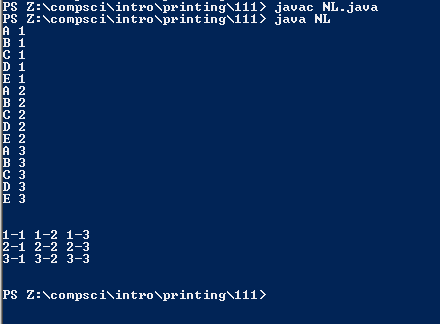# 111

## Code

```    ///Name: Joshua Bautista
///Period: 6
///Date: 6/4/16

public class NL
{
public static void main(String[] args)
{
// this is #1 - I'll call it "CN"
for ( int n=1; n <= 3; n++ )
{
for ( char c='A'; c <= 'E'; c++  )
{
System.out.println( n + " " + c );
}
}

System.out.println("\n");

// this is #2 - I'll call it "AB"
for ( int a=1; a <= 3; a++ )
{
for ( int b=1; b <= 3; b++ )
{
System.out.print( a + "-" + b + " " );
}
System.out.println("");
}

System.out.println("\n");

}
}

///the variable n changes due to n changing 3 times every time c changes
///the output changes by counting the number then charater, not character then number
///changes by displaying the number pairs vetically not than horizontally
///changes by making it a new line everytime b counts to 3 and everytime a change
```

### Picture of the output